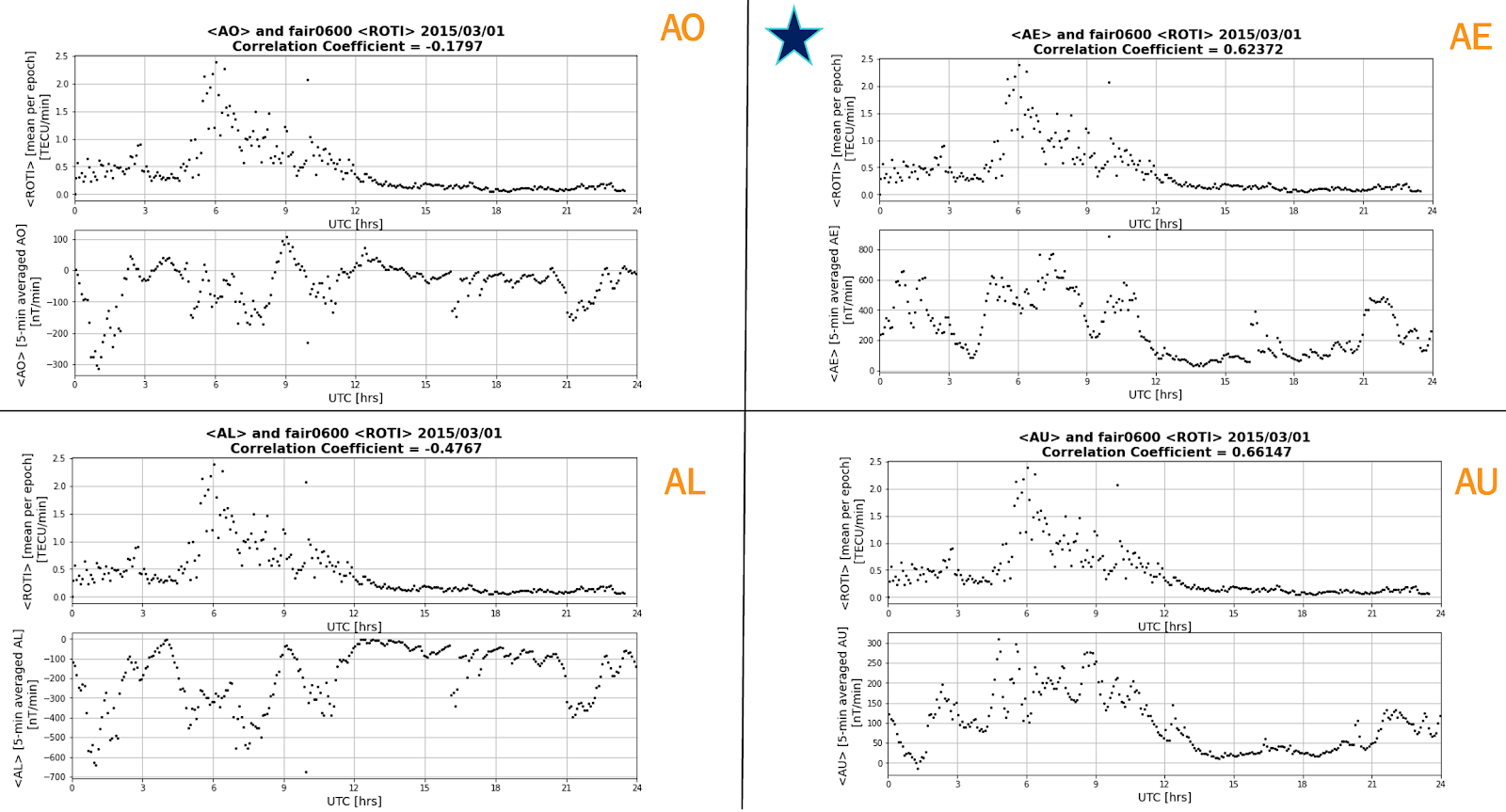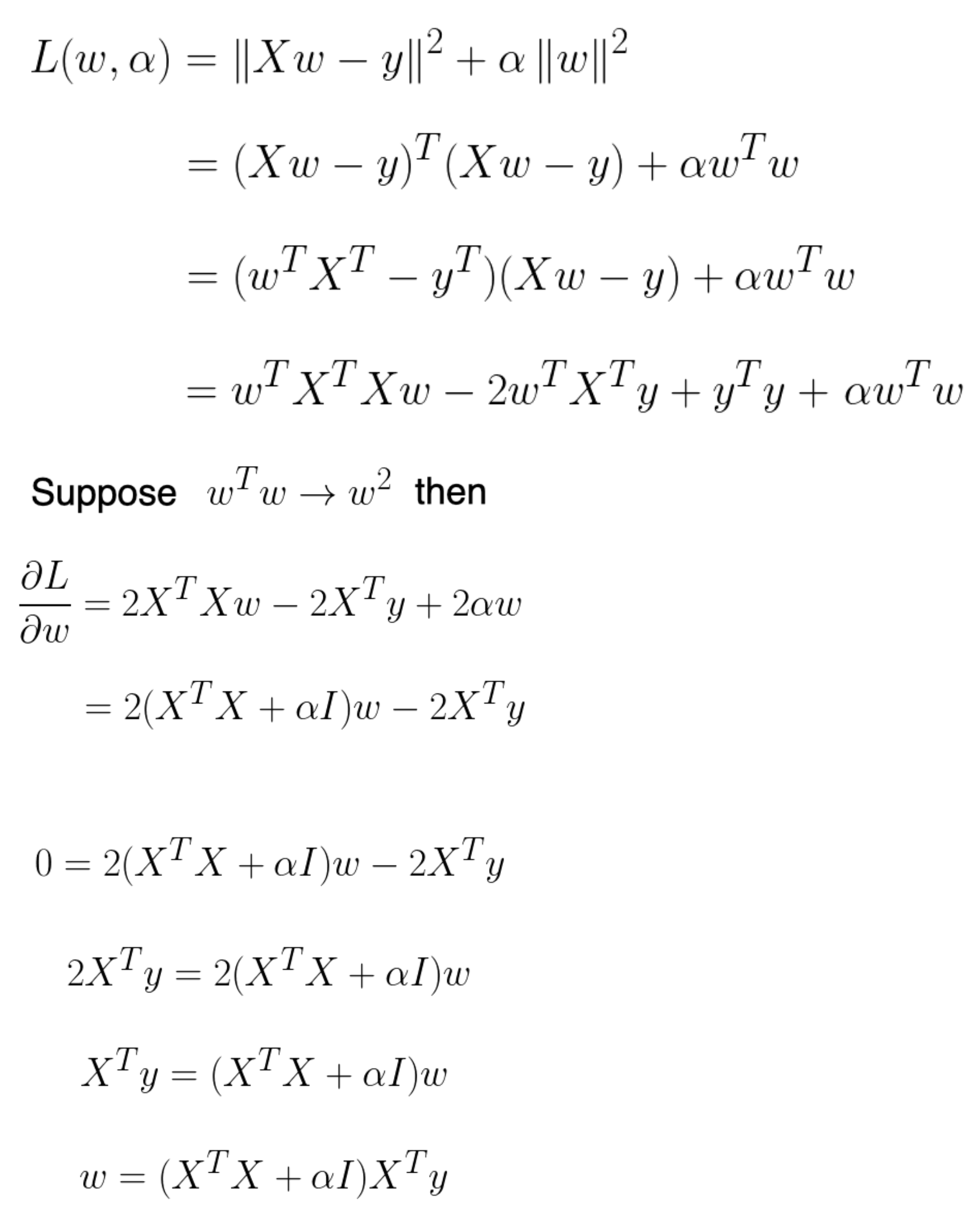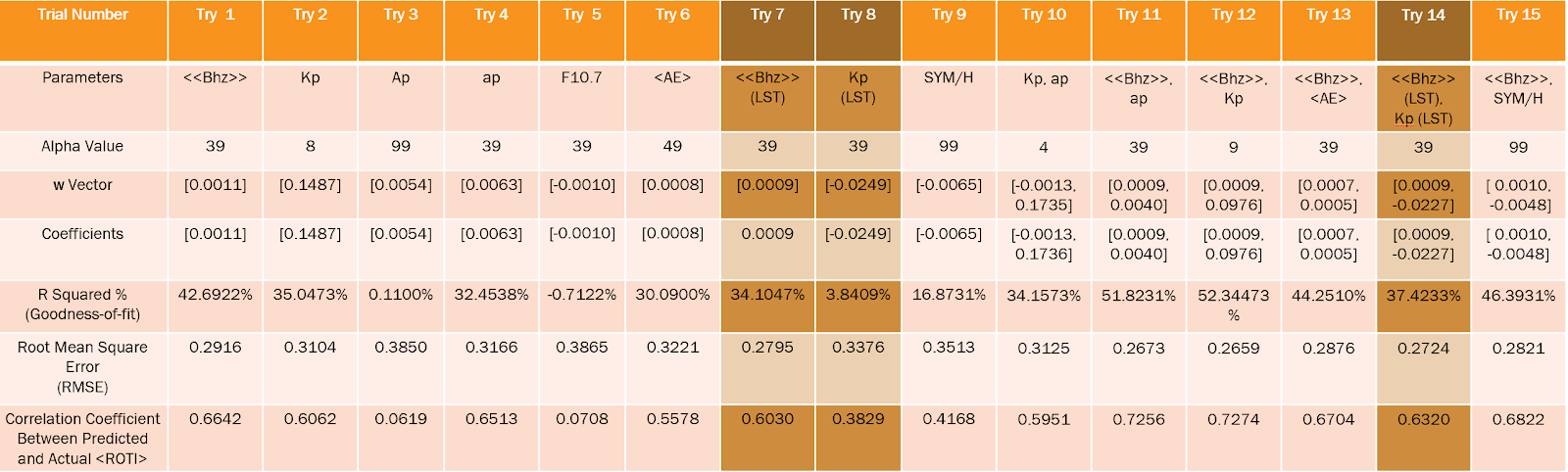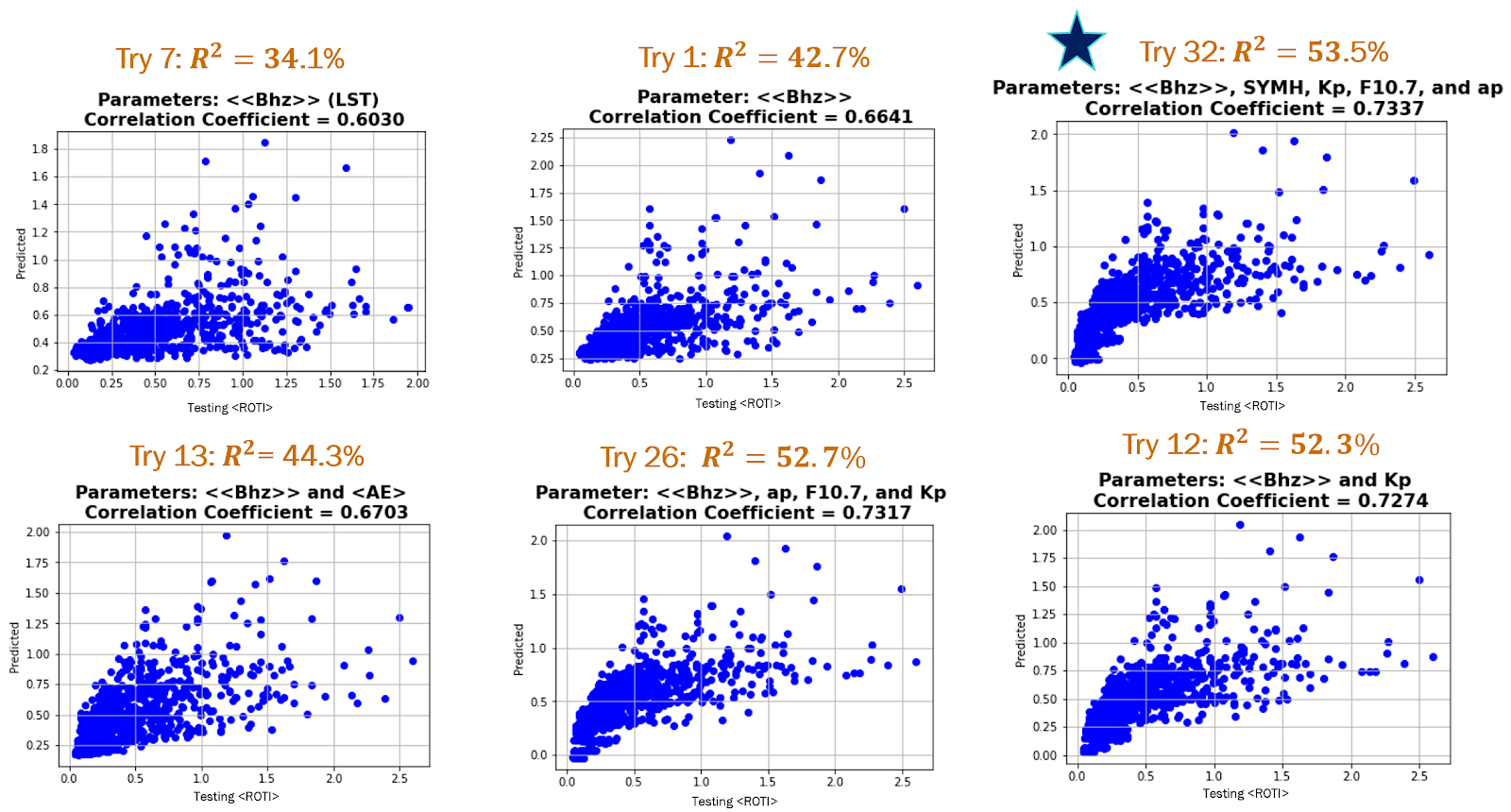Applying Machine-Learning to Predict High Latitude Alaskan Ionospheric Irregularities

Author: Annabel Gomez
Mentor: Dr. Xiaoqing Pi
Editor: Suchitra Dara

Introduction

Solar activities, such as solar flares and coronal mass ejections, produce perturbations in solar wind and the interplanetary magnetic field (IMF). These perturbations interact with the Earth’s magnetosphere, resulting in geomagnetic storms, charged particle precipitation, and plasma convection in the high-latitude ionosphere. These events can produce various ionospheric disturbances depending on location, geomagnetic conditions, presence of ionospheric currents, plasma convection, etc. The ionospheric disturbances, including the process of plasma instabilities, give rise to irregular structures in ionospheric density distribution, also known as ionospheric irregularities.Figure 1: TEC, ROT, and ROTI derived from dual frequency GPS phase data collected at Yellowknife, Canada, during May 16, 1995. The red color indicates the projected locations of observed ionospheric irregularities .

The Wide Area Augmentation System (WAAS) was developed by the Federal Aviation Administration (FAA) of the United States to support aviation navigation . WAAS provides a ground-based global positioning system (GPS) tracking network, master stations for data processing, and geostationary satellites to relay WAAS data. To increase navigation accuracy, WAAS generates ionospheric correction data and broadcasts it to its users. Ionospheric irregularities cause scintillation of GPS signal power and phase, which can interrupt radio signal reception and degrade the integrity and accuracy of technology applications that rely on the Global Navigation Satellite System (GNSS).

Ionospheric irregularities can be explained and measured as described by Figure 1. The progression of rate of total electron content (TEC) is measured as the rate of TEC (ROT). Both ROT and rate of TEC index (ROTI) are measurements of ionospheric irregularities that can cause L-band radio signal scintillations; the ROTI index is the standard deviation of ROT . A value of ROTI at or near zero represents a low level of disturbances, while a high value represents the presence of ionospheric irregularities indicating a stormy period.

The goal of this research is to investigate which space weather and geophysical measurements are useful in machine-learning (ML) techniques for a prediction system that uses historical GPS data to predict the location, time, and intensity of concerning irregularities. The system is targeted to reduce the impact of scintillation effects on GNSS data and applications.

Correlation Analyses

The ionospheric data used for this analysis was ROTI data with 5-minute intervals. The ROTI data was obtained by processing GPS phase data collected at Fairbanks, Alaska (station name: FAIR, station coordinates: 64.98º geographic latitude and 212.5º geographic longitude). At each epoch, <ROTI> is obtained by averaging ROTI values over a number of satellites in view.

The first main parameter used was auroral electrojet ((AE)) indices derived from longitudinally distributed magnetic field data in the polar region. This data includes four indices:

• AU: The upper envelope expressing the strongest intensity of eastward auroral electrojets.
• AL: The lower envelope expressing the strongest intensity of westward auroral electrojets.
• AE:$AU - AL$
• AO:$\frac{(AU + AL)}{2}$

A correlation analysis was first conducted between the ROTI and (AE) data. On average, the <AE> index, averaged over 5-minute intervals, had a higher correlation with <ROTI>, though <AU> occasionally correlated more with <ROTI> such as the case for March 01, 2015 (Figure 2). In general, there were more complications with <AL> and <AO> indices, as is evident with the negative values in contrast to positive <ROTI> values. Additionally, while <AE> did correlate more with <ROTI>, the correlation coefficient was 0.63, indicating that there were periods of time where <AE> data was disturbed and <ROTI> was not. Furthermore, from Figure 3, it is apparent that the relationship between <AE> and ROTI is non-linear. Thus (AE) data is not ideal for forming <ROTI> predictions, since it is measured along many longitudes (twelve to be exact) while ROTI measures localized irregularities.Figure 2: Each of the averaged 4 indices of (AE) data against universal time and data against universal time for March 01, 2015.

Since (AE) did not correlate well with <ROTI>, new data were needed. Localized magnetometer data, provided by the SuperMag Organization, was the next main parameter used. This data was collected at the Alaskan station of College (station name: CMO, station coordinates: 64.87º geographic latitude and 212.14º geographic longitude). To obtain a better correlation with <ROTI>, a combination of the magnetic field’s horizontal and vertical components was calculated and used: First, Bh =$\sqrt{Bn^{2} + Be^{2}}$, was computed where Bn and Be are the northern and eastern components of the magnetic field, respectively. Then, Bhz = Bh – Bz was determined to finally quantify <<Bhz>> = 5-minute average of Bhz for 24 hours, starting at 2.5 minutes Universal Time Coordinated (UTC).

Additionally, several other measurements, such as solar radio flux, solar wind, IMF components, and geomagnetic field indices, were used for analysis, as shown below in Table 1.

Table 1: Parameters and Measurements

All the data used, including <<Bhz>> and <ROTI>, are measured in UTC. Tests were also conducted to determine if the correlation improves when data is compared at Local Solar Time using the following formula:$LST = UTC[hours] + \frac{longitude[degrees]}{15}$

Using magnetometer data collected at the same longitude as the ROTI data gave an improved correlation. In fact, looking at the same day, March 01, 2015, the correlation between <<Bhz>> and <ROTI> is 0.82, an increase of 0.2 compared to using (AE) data. Referring to Figure 4, the periods of disturbed and undisturbed times match for both <<Bhz>> and <ROTI>. Additionally, Figure 5 shows a more linear relationship between <<Bhz>> and <ROTI> in comparison to that between (AE) and <ROTI>.

Machine-Learning and Ridge RegressionsFigure 6: 1. High variance in contrast to low variance where variance is related to a model failing to fit the testing set . 2. High bias in contrast to low bias where bias is related to a model failing to fit the training set .3. The contrast between an under fitted, balanced, and overfit model .

Three integral components of a ML algorithm are variance, bias (regularization), and overfitting, as seen in Figure 6 . Variance is a measure of how accurately a model can change when presented with a different data set. High variance is associated with a model failing to fit the testing dataset. Bias is the inability of a method to capture the true relationship of data. Typically, bias is used to develop a model that can predict targets for data that follow a general pattern, rather than a specific one. Bias is associated with a model failing to fit the training set of data. Finally, overfitting occurs when a polynomial with a higher degree than what is needed is used to model data. Overfitting causes a model to perform well for training samples but to fail when it comes to generalizing.Figure 7: Variance and bias have a trade-off relationship with model complexity. This means that a simple model has a high bias and low variance, and vice versa. The OLS model is at the far right (high complexity). With added regularization, the OLS model can move to the sweet spot in the middle where the error is at its lowest .

The algorithm used to produce <ROTI> predictions is a Ridge Regression. A Ridge Regression is a Linear Regression (sum of squares) with a small bias . This results in a significant drop in variance. However, starting with a slightly deficient fit results in an improved long-term prediction since the same pattern that the model was trained on is not memorized. A Ridge Regression is ideal for working with data that shows multicollinearity tendencies, such as <ROTI> data. In comparison, an Ordinary Least Squares (OLS) model treats all variables equally and is unbiased. The OLS model therefore becomes more complex as the number of variables increases. As displayed in Figure 7, the optimal model complexity is at the middle of the plot, where the error is at its lowest. Unlike the OLS model, the regularization parameter of a Ridge Regression can be tuned to change the model’s coefficients and produce more accurate predictions.

The Ridge Regression has several variables and parameters:

• α = Hyperparameter that determines the regularization strength. The larger the alpha value, the stronger the regularization. A large alpha increases the bias of the model significantly. An alpha value equal to 1 is equivalent to a Linear Regression.
• w = Vector of coefficients
• B = Regression coefficients to be estimated
• e = Error residuals
• X = Matrix of independent variables (x)
• Y = Dependent variable (prediction)

The Ridge Regression equation, in matrix form, used to calculate desired predictions is shown in (1) .

Y = XB + e                                  (1)

The loss function of the Ridge Regression is the linear least squares function, as shown in (2), and the regularization is given by the L2 norm, which calculates the distance of a vector coordinate from the origin of the vector in space. It is equivalent to the OLS model plus α multiplied by the summation of the squared coefficient values .$Lf = \sum_{i}^{n}(y_{pred}-y)^2+\alpha\omega_0^2+ \alpha \omega_ 1^2+ \alpha \omega_ 2^2+ \alpha \omega_ 4^2...$ (2)

Overall, when calculating predictions, the Ridge Regression aims to minimize the loss function by finding a balance between minimizing the residual sum of squares as well as the coefficients (3) .$\sum_{i}^{n} (y_{pred}-y)^2 = \sum_{i}^{n} (\omega x-y)^2$                (3)

Coefficients of the Ridge Regression are calculated by first taking the cost function, performing some algebra, taking the partial derivative of the cost function with respect to w, and setting it equal to 0 to solve (4) .(4)

The Ridge Regression model used to predict <ROTI> was programmed using Python and three main libraries: Pandas, Numpy, and Scikit-Learn. It was fit and tested on data from a total of 22 disturbed and stormy days with 18 days used for training and 4 days used for testing. The overall goal was to test which space weather and geophysical measurements would be useful for a ML prediction system. The test was performed with historical GPS and magnetometer data to predict the location, time, and intensity of concerned irregularities, under various geophysical and space weather conditions.

Two measures of performance were used to determine the accuracy of the Ridge Regression model: Root Mean Square Error (RMSE) and R squared (R2). RMSE is the average magnitude of the residuals, or error, and is represented by (5). It is optimal to obtain an RMSE as close to 0 as possible .$rmse = \sqrt{mean((Predicted - Actual)^2)}$       (5)$R^2$ represents the proportion of variance for a target variable, explained by the independent variables, and is expressed as a percentage. It can be represented by (6), where$u$ is the sum of residual squares,$u = sum (Actual - Predicted)^2$, and$v$ is the sum of squares of the measurement deviation from the mean,$v = sum(Actual - mean(Actual))^2$. For our standards, an$R^2$ value between 30% and 50% is considered weak, an$R^2$ value between 50% and 70% is considered moderate, and an$R^2$ value greater than 70% is considered strong .$R^2 = (1 -\frac{u}{v})$                            (6)

Experimental Results

As shown in Tables 2-4, many parameters and combinations of parameters were tested using a Ridge Regression model. The outputs of the Regression process include an ideal α value, the w vector, the Ridge Regression coefficients, the$R^2$, RMSE value, and the correlation coefficient between the predicted and actual <ROTI> for the specific group of inputs.

Table 2: Ridge Regression Results (Part 1/3)Table 2: Ridge Regression Results with a single input parameter (Trials 1-9) and two input parameters (Trials 10-15). The columns in brown represent the parameters of data tested in LST as well as their correlation against converted to LST.

Table 4: Ridge Regression Results (Part 3/3)Table 4: Ridge Regression Results with four or more input parameters. The column in blue represents the combination of parameters that resulted in the largest value and correlation coefficient between the predicted and actual .

The combination of parameters with the highest correlation coefficient and$R^2$ value was <<Bhz>>, SYM/H, Kp, ap, and F10.7 (all in UT time) (Trial 32). The most impactful parameters were <<Bhz>> and Kp. The increase in$R^2$ from Trial 1, where <<Bhz>> was a single parameter, to Trial 12, where both <<Bhz>> and Kp were parameters, was ~9.7%. It is also evident in Figure 8 that the scattering of the predicted vs. actual ROTI plot became tighter than that of the other plots. It was observed that the parameters converted to LST had lower correlations with <ROTI> than those in UTC. For example, the$R^2$ value in Trial 7, where <<Bhz>> was in LST, decreased by ~8.6% in comparison to Trial 1 where <<Bhz>> was in UT. Additionally, <AE> was used as a parameter in Trials 6, 13, 16, 22, 23, 25, 30, 31, and 33, and it was clear that the regression with (AE) performed somewhat worse for predictions of irregularities. This may be because (AE) is a longitudinally averaged index while the irregularities are localized.Figure 8: The depiction of the results in Tables 2-4 as scatter plots between the tested and predicted .

Conclusions

Since the largest$R^2$ value obtained from the Ridge Regression predictions was 53.5%, the accuracy of the predictions is not ideal. There are several reasons why this may have occurred. To begin with, the data samples were relatively small, since only 18 days’ worth of data samples were used to train the model. However, this was intentional; the main objective was to experiment which parameters and combinations thereof correlated best with <ROTI>. A different model may have been more appropriate, but the Ridge Regression was selected since it allowed an easy transition between various inputs. It is important to note that the parameters selected were all space weather and geomagnetic measurements, while the irregularities may be driven more directly by other nonlinear processes, contrasting the Ridge Regression’s linear approach.

Understanding how and why these different physical phenomena are correlated is crucial in making accurate predictions. As the development of the ML algorithm continues, its output will aid and contribute to the representation and understanding of the variations of the ionospheric state. The goal for the algorithm, once it is implemented, is to predict the location, time, and intensities of ionospheric irregularities under various geophysical and space weather conditions, reducing the impact of ionospheric scintillation on receptions of GNSS signals and data and improving navigation systems.

Acknowledgements

The author would like to thank Dr. Xiaoqing Pi for providing research guidance, technical assistance, and for processing and providing the ROTI data; Caltech Northern California Associates for the fellowship; Peyman Tavallali, Jet Propulsion Laboratory, California Institute of Technology, for suggesting the use of the Ridge Regression Algorithm; IGS for the management of the GNSS Data Archives; and SuperMag Organization for the distribution of magnetometer data.

References

 Pi, X., A. J. Mannucci, U. J. Lindqwister, and C. M. Ho, Monitoring of global ionospheric irregularities using the worldwide GPS network, Geophys. Res. Lett., 24, 2283, 1997.

 Pi, X., Mannucci, A.J., Valant-Spaight, B., Bar-Sever, Y., Romans, L. J., Skone, S., Sparks, L., Hall, G. Martin, “Observations of Global and Regional Ionospheric Irregularities and Scintillation Using GNSS Tracking Networks,” Proceedings of the ION 2013 Pacific PNT Meeting, Honolulu, Hawaii, April 2013, pp. 752-761.

 Maklin, C. (2019, August 19). Ridge Regression Python Example. Retrieved from https://towardsdatascience.com/ridge-regression-python-example-f015345d936b

 Qshick. (2019, January 03). Ridge Regression for Better Usage. Retrieved from https://towardsdatascience.com/ridge-regression-for-better-usage-2f19b3a202db

 Chapter 335 Ridge Regression – NCSS .Chapter 335 Ridge Regression Introduction Ridge Regression is – [PDF Document]. (n.d.). Retrieved from https://fdocuments.in/document/chapter-335-ridge-regression-ncss-chapter-335-ridge-regression-introduction.html